Information submitted through the support site is private but is not hosted within your secure CDD Vault. Please do not include sensitive intellectual property in your support requests.

# Chemical Properties Calculated by CDD Vault During Chemical Registration

Users may register new Molecules into CDD Vault either manually, one-at-a-time, through the interface or by using the Data Import wizard. Whichever mechanism is used, the chemical properties described below are automatically calculated by CDD Vault for every chemical structure registered.

Lipinski Properties

Molecular weight
The average molecular mass calculated from the standard atomic weights, averaged over all isotopes weighted by their natural abundance.

log P
The logarithm of the partition coefficient. The implementation uses the approach described in Gedeck et al. (DOI: 10.1021/acs.jcim.7b00315) using publicly available log P data. Learn more…

H-bond donors and H-bond acceptors
The number of hydrogen bond donors/acceptors in this molecule.

Lipinski Rule of Five
A rule of thumb to evaluate whether a chemical molecule would likely be an orally active drug in humans. The rule is not predictive, but it is a widely adopted, useful guideline. The rule is composed of four criteria, all based on numbers that are multiples of the number five:

1. Molecular weight < 500 g/mol
2. log P less than 5
3. No more than 5 hydrogen bond donors
4. No more than 10 hydrogen bond acceptors

If four criteria are satisfied, CDD displays the text "Satisfied". If three are satisfied, CDD displays the text "One Violation". If fewer than three are satisfied, CDD displays the text "Violated". Technically the rule is violated if there are any violations, however sometimes there is value in distinguishing between one violation and more than one violation. For more information, see Lipinski et al.

log D
The logarithm of the distribution coefficient at pH 7.4. The distribution coefficient is the ratio of the sum of the concentrations of all species of the molecule in octanol to the sum of the concentrations of all species of the molecule in water. The implementation is based on work by Gedeck et al. (DOI: 10.1021/acs.jcim.7b00315) using log D data deposited by Astra Zeneca into ChEMBL (DOI: 10.6019/CHEMBL3301361). Learn more…

log S
The aqueous solubility at pH 7.4. The solubility of a molecule is the concentration in equilibrium with the solid phase that is dissolved into the solution. The implementation is based on the following publication.
ESOL: Estimating Aqueous Solubility Directly from Molecular Structure
John S. Delaney, J. Chem. Inf. Comput. Sci., 2004, 44, 1000 - 1005.
The parameters for ESOL were refitted using multiple linear regression.

pKa
The implementation is based on work of Lu et al. (DOI: 10.1021/acs.jcim.9b00498) using experimental data from Mansouri et al. (DOI: 10.1186/s13321-019-0384-1). The value reported is the strongest pKa, either basic or acidic. When the pH of the solution is equal with pKa, the concentrations of dissociated and undissociated species are equal. Learn more…

Topological Polar Surface Area
The topological polar surface area (TPSA) is formed by polar atoms of a molecule. It is a descriptor that shows good correlation with passive molecular transport through membranes, and so it is used to estimate drug transport properties. Estimation of the TPSA is based on the method given in Ertl et al.

Exact mass
The monoisotopic mass calculated from the weights of the most abundant natural isotopes of the elements.

Fsp3
Number of sp3 hybridized carbons divided by the total carbon count.

Heavy atom count
The total number of atoms in the molecule excluding hydrogen.

Rotatable bonds
The number of rotatable bonds reflects molecular flexibility and has been shown to correlate with the oral bio-availability of a drug. Unsaturated bonds, and single bonds connected to hydrogens or terminal atoms, single bonds of amides, sulfonamides and those connecting two hindered aromatic rings (having at least three ortho substituents) are considered non-rotatable.

Formula
The chemical formula of the molecule according to the Hill system: the number of carbon atoms is indicated first, the number of hydrogen atoms next (including deuterium and tritium when using D and T symbols), and then the number of all other chemical elements subsequently, in alphabetical order.

Composition
The elemental composition (CHNO Analysis) given in weight percentage (w/w %) calculated from the atomic masses.

CNS MPO score
CDD's implementation of the CNS MPO score is based on six physicochemical properties and is meant to help prioritize CNS design candidates and predict the potential success of these compounds. The CNS MPO score was first introduced in this publication, and our implementation is based on this paper.

CDD Vault calculates the CNS MPO score using the same linear transformational functions and inflection points described in Wager et al. (2010) mentioned above, but uses physicochemical properties provided by the CDD Chemistry tools. There are six properties that are used as inputs, and they contribute to the score as follows:

LogP

• calculated using logP function
• LogP values ≤ 3 contribute 1.0 to the score
• LogP values > than 5 contribute nothing
• LogP values between these inflection points contribute based on a linear function.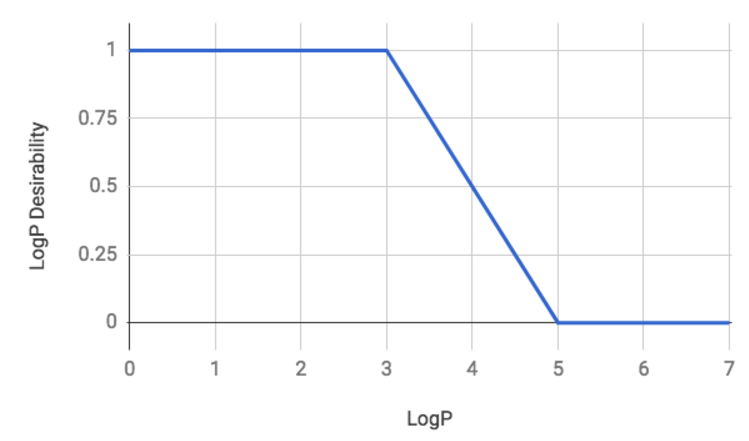LogD

• calculated using logD('7.4') function
• LogD values ≤ 2 contributes 1.0 to the score
• LogD values > 4 contribute nothing
• LogD values between these inflection points contribute based on a linear functionMolecular Weight

• Molecular weight of the neutralized core structure is calculated by CDD Chemistry tools
• Molecular weight values ≤ 360 contribute 1.0 to the score
• Molecular weight values > 500 contribute nothing
• Molecular weight values between these inflection points contribute based on a linear function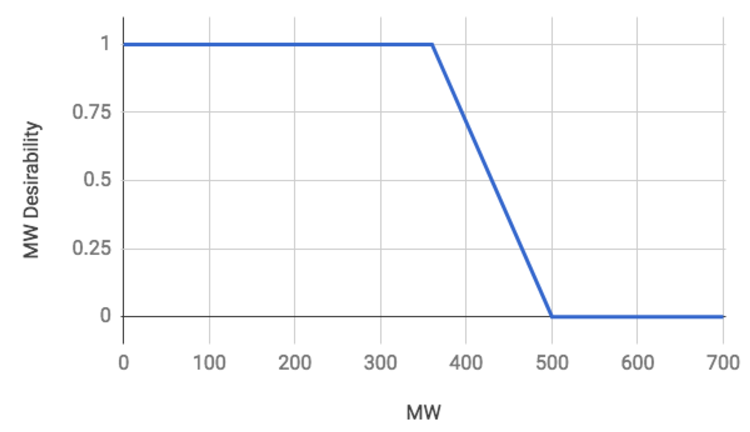TPSA

• Topological polar surface area is estimated based on the method given in Ertl et al.
• TPSA values between 40 and 90 contribute 1.0 to the score
• TPSA values ≤ 20 or > 120 contribute nothing
• TPSA values between the two pairs of inflection points contribute based on linear functions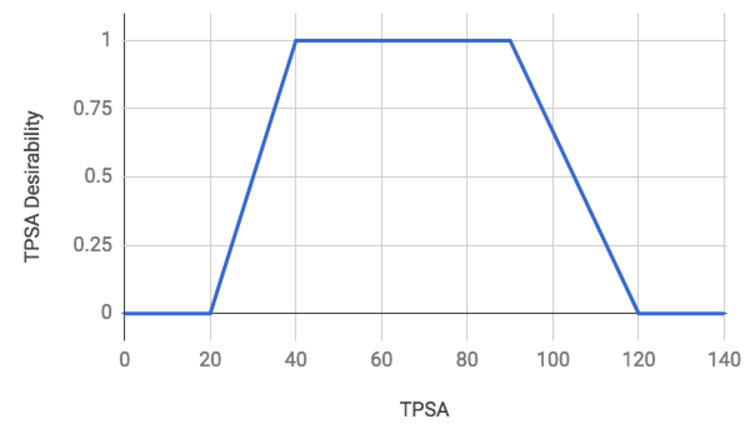HBD

• The number of hydrogen bond donors is calculated using CDD Chemistry tools
• HBD counts ≤ 0.5 contribute 1.0 to the score
• HBD counts > 3.5 contribute nothing
• HBD counts between these inflection points contribute based on a linear function.

Note:

Figure 1 in Wager et al. (2016) lists the inflection points as 0 and 4, but Table 2 in the same paper clearly shows that 0.5 and 3.5 are being used for the calculation.

Wager et al. (2010) lists the inflection points as 0.5 and 3.5.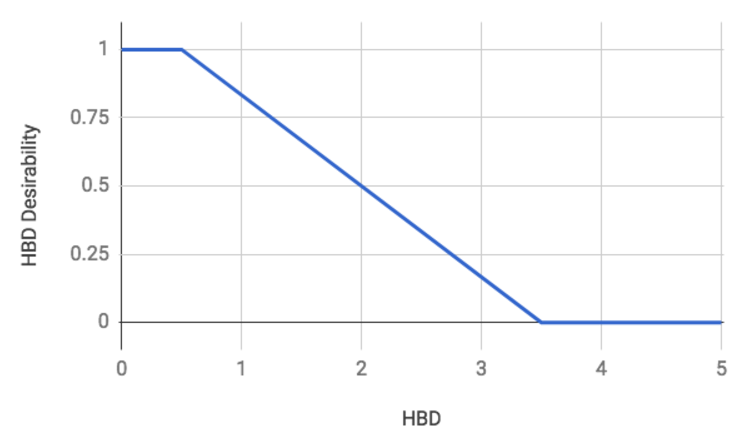pKa

• The most basic pKa is calculated using the CDD Chemistry tools
• pKa values ≤ 8 contribute 1 to the score
• pKa values > 10 contribute nothing
• pKa values between these inflection points contribute based on a linear function
• structures for which no basic pKa can be calculated are treated as if the pKa was ≤ 8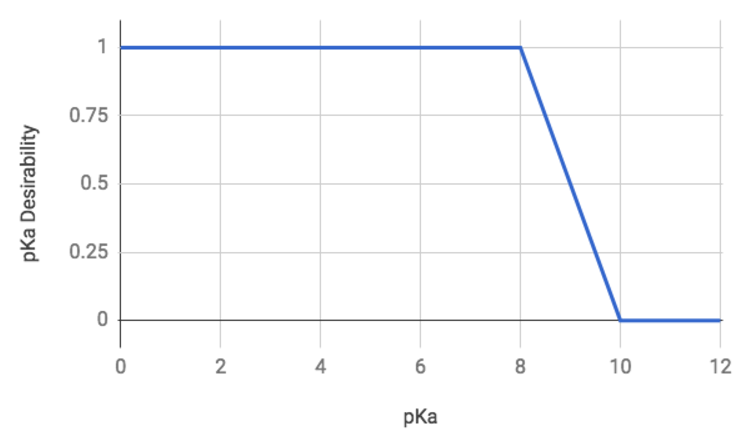These 6 individual contributions (log P, log D, MW, TPSA, HBD, pKa) are summed to produce the final CNS MPO score shown in CDD Vault.